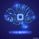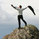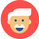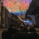한국어
TradingView
26010 뷰
26010
This is version 2 of my ANN strategy. This version will not repaint, but requires some settings configuration.
The reason why the old version repainted, is because it used the daily OHLC/4 which kept changing, causing lower timeframes ro repaint.
You can now specify which timeframes to use. The bigger timeframe is set in the settings (e.g. 1 day). You set the smaller settings by opening a certain chart (e.g. a 4h chart).
The timeframes are used to calculate the percentual difference, which is fed to the ANN as input

Link to version 1:
```//@version=2
strategy("ANN Strategy v2")

threshold = input(title="Threshold", type=float, defval=0.0000, step=0.0001)
timeframe = input(title="Timeframe", type=resolution, defval='1D' )

getDiff() =>
yesterday=security(tickerid, timeframe, ohlc4)
today=ohlc4
delta=today-yesterday
percentage=delta/yesterday

PineActivationFunctionLinear(v) => v
PineActivationFunctionTanh(v) => (exp(v) - exp(-v))/(exp(v) + exp(-v))

l0_0 = PineActivationFunctionLinear(getDiff())

l1_0 = PineActivationFunctionTanh(l0_0*0.8446488687)
l1_1 = PineActivationFunctionTanh(l0_0*-0.5674069006)
l1_2 = PineActivationFunctionTanh(l0_0*0.8676766445)
l1_3 = PineActivationFunctionTanh(l0_0*0.5200611473)
l1_4 = PineActivationFunctionTanh(l0_0*-0.2215499554)

l2_0 = PineActivationFunctionTanh(l1_0*0.3341657935 + l1_1*-2.0060003664 + l1_2*0.8606354375 + l1_3*0.9184846912 + l1_4*-0.8531172267)
l2_1 = PineActivationFunctionTanh(l1_0*-0.0394076437 + l1_1*-0.4720374911 + l1_2*0.2900968524 + l1_3*1.0653326022 + l1_4*0.3000188806)
l2_2 = PineActivationFunctionTanh(l1_0*-0.559307785 + l1_1*-0.9353655177 + l1_2*1.2133832962 + l1_3*0.1952686024 + l1_4*0.8552068166)
l2_3 = PineActivationFunctionTanh(l1_0*-0.4293220754 + l1_1*0.8484259409 + l1_2*-0.7154087313 + l1_3*0.1102971055 + l1_4*0.2279392724)
l2_4 = PineActivationFunctionTanh(l1_0*0.9111779155 + l1_1*0.2801691115 + l1_2*0.0039982713 + l1_3*-0.5648257117 + l1_4*0.3281705155)
l2_5 = PineActivationFunctionTanh(l1_0*-0.2963954503 + l1_1*0.4046532178 + l1_2*0.2460580977 + l1_3*0.6608675819 + l1_4*-0.8732022547)
l2_6 = PineActivationFunctionTanh(l1_0*0.8810811932 + l1_1*0.6903706878 + l1_2*-0.5953059103 + l1_3*-0.3084040686 + l1_4*-0.4038498853)
l2_7 = PineActivationFunctionTanh(l1_0*-0.5687101164 + l1_1*0.2736758588 + l1_2*-0.2217360382 + l1_3*0.8742950972 + l1_4*0.2997583987)
l2_8 = PineActivationFunctionTanh(l1_0*0.0708459913 + l1_1*0.8221730616 + l1_2*-0.7213265567 + l1_3*-0.3810462836 + l1_4*0.0503867753)
l2_9 = PineActivationFunctionTanh(l1_0*0.4880140595 + l1_1*0.9466627196 + l1_2*1.0163097961 + l1_3*-0.9500386514 + l1_4*-0.6341709382)
l2_10 = PineActivationFunctionTanh(l1_0*1.3402207103 + l1_1*0.0013395288 + l1_2*3.4813009133 + l1_3*-0.8636814677 + l1_4*41.3171047132)
l2_11 = PineActivationFunctionTanh(l1_0*1.2388217292 + l1_1*-0.6520886912 + l1_2*0.3508321737 + l1_3*0.6640560714 + l1_4*1.5936220597)
l2_12 = PineActivationFunctionTanh(l1_0*-0.1800525171 + l1_1*-0.2620989752 + l1_2*0.056675277 + l1_3*-0.5045395315 + l1_4*0.2732553554)
l2_13 = PineActivationFunctionTanh(l1_0*-0.7776331454 + l1_1*0.1895231137 + l1_2*0.5384918862 + l1_3*0.093711904 + l1_4*-0.3725627758)
l2_14 = PineActivationFunctionTanh(l1_0*-0.3181583022 + l1_1*0.2467979854 + l1_2*0.4341718676 + l1_3*-0.7277619935 + l1_4*0.1799381758)
l2_15 = PineActivationFunctionTanh(l1_0*-0.5558227731 + l1_1*0.3666152536 + l1_2*0.1538243225 + l1_3*-0.8915928174 + l1_4*-0.7659355684)
l2_16 = PineActivationFunctionTanh(l1_0*0.6111516061 + l1_1*-0.5459495224 + l1_2*-0.5724238425 + l1_3*-0.8553500765 + l1_4*-0.8696190472)
l2_17 = PineActivationFunctionTanh(l1_0*0.6843667454 + l1_1*0.408652181 + l1_2*-0.8830470112 + l1_3*-0.8602324935 + l1_4*0.1135462621)
l2_18 = PineActivationFunctionTanh(l1_0*-0.1569048216 + l1_1*-1.4643247888 + l1_2*0.5557152813 + l1_3*1.0482791924 + l1_4*1.4523116833)
l2_19 = PineActivationFunctionTanh(l1_0*0.5207514017 + l1_1*-0.2734444192 + l1_2*-0.3328660936 + l1_3*-0.7941515963 + l1_4*-0.3536051491)
l2_20 = PineActivationFunctionTanh(l1_0*-0.4097807954 + l1_1*0.3198619826 + l1_2*0.461681627 + l1_3*-0.1135575498 + l1_4*0.7103339851)
l2_21 = PineActivationFunctionTanh(l1_0*-0.8725014237 + l1_1*-1.0312091401 + l1_2*0.2267643037 + l1_3*-0.6814258121 + l1_4*0.7524828703)
l2_22 = PineActivationFunctionTanh(l1_0*-0.3986855003 + l1_1*0.4962556631 + l1_2*-0.7330224516 + l1_3*0.7355772164 + l1_4*0.3180141739)
l2_23 = PineActivationFunctionTanh(l1_0*-1.083080442 + l1_1*1.8752543187 + l1_2*0.3623326265 + l1_3*-0.348145191 + l1_4*0.1977935038)
l2_24 = PineActivationFunctionTanh(l1_0*-0.0291290625 + l1_1*0.0612906199 + l1_2*0.1219696687 + l1_3*-1.0273685429 + l1_4*0.0872219768)
l2_25 = PineActivationFunctionTanh(l1_0*0.931791094 + l1_1*-0.313753684 + l1_2*-0.3028724837 + l1_3*0.7387076712 + l1_4*0.3806140391)
l2_26 = PineActivationFunctionTanh(l1_0*0.2630619402 + l1_1*-1.9827996702 + l1_2*-0.7741413496 + l1_3*0.1262957444 + l1_4*0.2248777886)
l2_27 = PineActivationFunctionTanh(l1_0*-0.2666322362 + l1_1*-1.124654664 + l1_2*0.7288282621 + l1_3*-0.1384289204 + l1_4*0.2395966188)
l2_28 = PineActivationFunctionTanh(l1_0*0.6611845175 + l1_1*0.0466048937 + l1_2*-0.1980999993 + l1_3*0.8152350927 + l1_4*0.0032723211)
l2_29 = PineActivationFunctionTanh(l1_0*-0.3150344751 + l1_1*0.1391754608 + l1_2*0.5462816249 + l1_3*-0.7952302364 + l1_4*-0.7520712378)
l2_30 = PineActivationFunctionTanh(l1_0*-0.0576916066 + l1_1*0.3678415302 + l1_2*0.6802537378 + l1_3*1.1437036331 + l1_4*-0.8637405666)
l2_31 = PineActivationFunctionTanh(l1_0*0.7016273068 + l1_1*0.3978601709 + l1_2*0.3157049654 + l1_3*-0.2528455662 + l1_4*-0.8614146703)
l2_32 = PineActivationFunctionTanh(l1_0*1.1741126834 + l1_1*-1.4046408959 + l1_2*1.2914477803 + l1_3*0.9904052964 + l1_4*-0.6980155826)

l3_0 = PineActivationFunctionTanh(l2_0*-0.1366382003 + l2_1*0.8161960822 + l2_2*-0.9458773183 + l2_3*0.4692969576 + l2_4*0.0126710629 + l2_5*-0.0403001012 + l2_6*-0.0116244898 + l2_7*-0.4874816289 + l2_8*-0.6392241448 + l2_9*-0.410338398 + l2_10*-0.1181027081 + l2_11*0.1075562037 + l2_12*-0.5948728252 + l2_13*0.5593677345 + l2_14*-0.3642935247 + l2_15*-0.2867603217 + l2_16*0.142250271 + l2_17*-0.0535698019 + l2_18*-0.034007685 + l2_19*-0.3594532426 + l2_20*0.2551095195 + l2_21*0.4214344983 + l2_22*0.8941621336 + l2_23*0.6283377368 + l2_24*-0.7138020667 + l2_25*-0.1426738249 + l2_26*0.172671223 + l2_27*0.0714824385 + l2_28*-0.3268182144 + l2_29*-0.0078989755 + l2_30*-0.2032828145 + l2_31*-0.0260631534 + l2_32*0.4918037012)

buying = l3_0 > 0 ? true : l3_0 < -0 ? false : buying

hline(0, title="base line")
//bgcolor(l3_0 > 0.0014 ? green : l3_0 < -0.0014 ? red : gray, transp=20)
bgcolor(buying ? green : red, transp=20)
plot(l3_0, color=silver, style=area, transp=75)
plot(l3_0, color=aqua, title="prediction")

longCondition = buying
if (longCondition)
strategy.entry("Long", strategy.long)

shortCondition = buying != true
if (shortCondition)
strategy.entry("Short", strategy.short)```@sirolf2009 hi
What you and many who called using strategy for today's ohlc4 called cheating don't understand is a very simple fact that makes your version 2 much worse than version 1 !!!

let me show that with a simple picture

The top chart is the daily chart and the one bellow is 30 min chart, now, the candle that i marked on the bottom chart is the last candle of that day ! take a look, where would be the OHLC4 price on that 30 min candle? compare it to what you would see on the daily chart !!!!

There is a 200\$ GAP in this situation !!!!

No matter what you would use for current candle's value calculation, in pine script, there will ALWAYS be repainting issue, that is a bad programming on the base structure of trading view ( they can deny it as much as they like ! ). But when you choose to call the current value on current candle, you can introduce HUGE error margin into the code just by doing it !!!!
응답This strategy does not work on the realtime
it has repaint ,
I would advise you to test my osilator (powerful bay signal) .
응답I'm very curious where you came up with the idea for this? Seems like such a random equation, would you mind sharing your thought process while coming up with this?
응답mych36
@mych36, A formula of this shape is an Array of a Neural Network
응답
KO 한국어
EN English
EN English (UK)
EN English (IN)
DE Deutsch
FR Français
ES Español
IT Italiano
PL Polski
SV Svenska
TR Türkçe
RU Русский
PT Português
ID Bahasa Indonesia
MS Bahasa Melayu
TH ภาษาไทย
VI Tiếng Việt
JA 日本語
ZH 简体中文
ZH 繁體中文
AR العربية
HE עברית